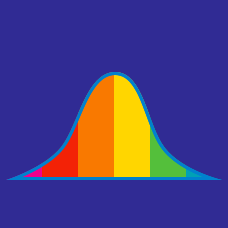Probability

# Effects of Changes to Data

A set of data points has a median of $45$. If we multiplied all the data points by $\frac{1}{5}$, what would be the new median?

A set of data points has a mean of $10$. If we multiplied all the data points by $2$, what would be the new mean?

A set of data points has a median of $16$. If we added $2$ to all the data points, what would be the new median?

A set of data points has a range of $10$. If we added $2$ to all the data points, what would be the new range?

A set of data points has a range of $12$. If we multiplied all the data points by $2$, what would be the new range?

×